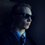# $1^\infty=2$

For any number $x$, we can express the difference between $x$ and $x$ raised to some power $n$ as:

$y=x-x^n$

To find which number $x$ most exceeds $x^n$, we can find the maximum point of the above function.

First the derivative:

$y\prime=1-nx^{(n-1)}$

And then the maximum (assuming that the power, $n$, is greater than 1):

$1-nx^{(n-1)}=0$

$nx^{(n-1)}=1$

$x^{(n-1)}=\frac{1}{n}$

$x=(\frac{1}{n})^{1/(n-1)}$

We can relabel this final equation as a function in $x$ and $y$ which will accept a power and yield the number which most exceeds itself raised to that power. In other words, a function which yields all the maximums of our original function ($y=x-x^n$).

$y=(\frac{1}{x})^{1/(x-1)}$

This function can be shown to converge to $1$:

$\lim_{x \to \infty}~(\frac{1}{x})^{1/(x-1)} = \lim_{x \to \infty}~(\frac{1}{x})^{0}=\lim_{x \to \infty}~1=\boxed{1}$

This is equivalent to saying that the number $n$ which most exceeds $n^{\infty}$ is $1$ ($1$ most exceeds $1^{\infty}$). Remember that (because it gets weirder!).

Now the function describing the difference between these "greatest" numbers and their respective powers is:

$y=(\frac{1}{x})^{1/(x-1)}-[(\frac{1}{x})^{1/(x-1)}]^x$

Simplifying:

$y=(\frac{1}{x})^{1/(x-1)}-[(\frac{1}{x})^{x/(x-1)}]$

$y=(\frac{1}{x})^{1/(x-1)}[1-(\frac{1}{x})^{(x-1)/(x-1)}]$

$y=(\frac{1}{x})^{1/(x-1)}[1-(\frac{1}{x})^{1}]$

$y=(\frac{1}{x})^{1/(x-1)}[1-(\frac{1}{x})]$

$y=(1-\frac{1}{x})(\frac{1}{x})^{1/(x-1)}$

This can also be shown to converge to $1$:

$\lim_{x \to \infty}~(1-\frac{1}{x})(\frac{1}{x})^{1/(x-1)} = \lim_{x \to \infty}~(1-0)(\frac{1}{x})^{0}=\lim_{x \to \infty}~1=\boxed{1}$

This is equivalent to saying that the difference between the number $n$ which most exceeds $n^{\infty}$ ($1$) and $n^{\infty}$ ($1^{\infty}$) is $1$.

This is understandably counter-intuitive, but taken in this context, it seems provable!

To finish, here is the grand consequence of this discussion:

$1^{\infty}-1=1$

Which means:

$\boxed{1^{\infty}=2}$

$\ddot\smile$Note by David Stiff
2 years, 1 month ago

This discussion board is a place to discuss our Daily Challenges and the math and science related to those challenges. Explanations are more than just a solution — they should explain the steps and thinking strategies that you used to obtain the solution. Comments should further the discussion of math and science.

When posting on Brilliant:

• Use the emojis to react to an explanation, whether you're congratulating a job well done , or just really confused .
• Ask specific questions about the challenge or the steps in somebody's explanation. Well-posed questions can add a lot to the discussion, but posting "I don't understand!" doesn't help anyone.
• Try to contribute something new to the discussion, whether it is an extension, generalization or other idea related to the challenge.

MarkdownAppears as
*italics* or _italics_ italics
**bold** or __bold__ bold
- bulleted- list
• bulleted
• list
1. numbered2. list
1. numbered
2. list
Note: you must add a full line of space before and after lists for them to show up correctly
paragraph 1paragraph 2

paragraph 1

paragraph 2

[example link](https://brilliant.org)example link
> This is a quote
This is a quote
    # I indented these lines
# 4 spaces, and now they show
# up as a code block.

print "hello world"
# I indented these lines
# 4 spaces, and now they show
# up as a code block.

print "hello world"
MathAppears as
Remember to wrap math in $$ ... $$ or $ ... $ to ensure proper formatting.
2 \times 3 $2 \times 3$
2^{34} $2^{34}$
a_{i-1} $a_{i-1}$
\frac{2}{3} $\frac{2}{3}$
\sqrt{2} $\sqrt{2}$
\sum_{i=1}^3 $\sum_{i=1}^3$
\sin \theta $\sin \theta$
\boxed{123} $\boxed{123}$

Sort by:

I think it depends on the “1”. Think of the context of e.

- 1 year, 11 months ago

I know, it really can't be taken literally. Reading this again months after I wrote it, I actually had a hard time following along! I think I had been inspired by something in my math textbook at the time.

- 1 year, 11 months ago

ooo

- 2 years ago

Actually I discovered an "error" in this absurdity which makes $1^{\infty}=2$. :)

- 1 year, 11 months ago